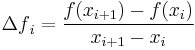# numpy Homework

Implement the following python functions. The name of the exercise should be the name of the function. You will need import numpy! On your own computer I recommend Anaconda because it is installed with numpy by default. Mind that you need the 2.7 version. Or you can use the leibniz.

## integral (2p)

The function should have 3 parameters, two real numbers and one integer:

  a: the left-hand-side of the interval
b: the right-hand-side of the interval
n: the number of equally spaced points in the partition, including a and b


a < b and n > 1.

The output should be a real number: the integral of the function sin(x) / x on the interval [a,b] calculated with the trapezoidal rule.

## derivative (3p)

The function should have 3 parameters, two real numbers and one integer:

  a: the left-hand-side of the interval
b: the right-hand-side of the interval
n: the number of uniformly distributed points in the interval


a < b and n > 1.

• The output should be a numpy vector of length n-1 containing the numerical derivative of the function sin(x) / x on the given interval using the random partition.
• The partition should contain n-2 uniformly distributed point in [a,b] plus x0 = a,xn − 1 = b.
• You can make this partition by making n-2 random points, sort them then add a to the front and b to the end.
• you might want to use: numpy.sort and numpy.random.rand

Remember the (forward) finite difference:${\Delta f}_i = \frac{f(x_{i+1})-f(x_i)}{x_{i+1}-x_i}$

## Handing-in

Send the exercises as a python code from your math email account to info1hazi@gmail.com

You should attach one python file containing the definition of the required functions without any test code or print command.

The file should be named:

  EN1_HF8_<user account>.py


the subject of the letter should be the same (without the extension). For me example:

  EN1_HF8_borbely.py